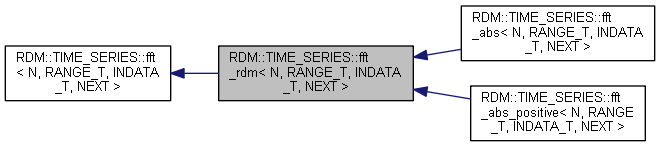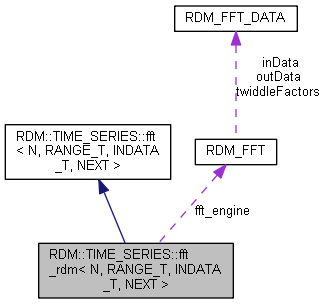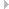RDM::TIME_SERIES::fft_rdm< N, RANGE_T, INDATA_T, NEXT > Class Template Reference

Template Class for doing an FFT using the RDM FFT implementation. More...

`#include "rdm_time_series_fft.h"`

Inheritance diagram for RDM::TIME_SERIES::fft_rdm< N, RANGE_T, INDATA_T, NEXT >:Collaboration diagram for RDM::TIME_SERIES::fft_rdm< N, RANGE_T, INDATA_T, NEXT >:## Protected Member Functions

RDM_RETCODE init (RDM_DB db)
Initialize this object. More...
fft_rdm ()
~fft_rdm ()Protected Member Functions inherited from RDM::TIME_SERIES::fft< N, RANGE_T, INDATA_T, NEXT >
virtual RDM_RETCODE doFft (void)=0
uint32_t init_tables_to_write_lock (RDM_TABLE_ID *tables)
ID of the tables where rows are inserted. More...
IDs of the tables where rows are read. More...
void unput (void)
Undo a previous put operation. More...
void unflush (void)
Undo a previous flush operation. More...
RDM_RETCODE init (RDM_DB db)
Initialize this object. More...

## Protected Attributes

RDM_FFT fft_engineProtected Attributes inherited from RDM::TIME_SERIES::fft< N, RANGE_T, INDATA_T, NEXT >
INDATA_T in_data [N]
RANGE_T aggregate_range
uint32_t nPublic Member Functions inherited from RDM::TIME_SERIES::fft< N, RANGE_T, INDATA_T, NEXT >
void reset (void)
Reset this object. More...
RDM_RETCODE flush_value (uint32_t threshold=1, RDM::DB::TRANSACTIONAL_T transactional=RDM::DB::NOT_TRANSACTIONAL)
Flush this object. More...
RDM_RETCODE flush_stats (uint32_t threshold=1, RDM::DB::TRANSACTIONAL_T transactional=RDM::DB::NOT_TRANSACTIONAL)
Flush this object. More...
template<class SOURCE_VALUE_T >
RDM_RETCODE put_value (SOURCE_VALUE_T *source_value, RDM::DB::TRANSACTIONAL_T transactional=RDM::DB::NOT_TRANSACTIONAL)
Template method for receiving a data value. More...
template<class SOURCE_STATS_T >
RDM_RETCODE put_stats (SOURCE_STATS_T *source_stats, RDM::DB::TRANSACTIONAL_T transactional=RDM::DB::NOT_TRANSACTIONAL)
Template method for receiving statistics. More...Static Protected Member Functions inherited from RDM::TIME_SERIES::fft< N, RANGE_T, INDATA_T, NEXT >
constexpr static int number_of_tables_to_write_lock (void)
Number of tables where rows are inserted. More...
Number of tables where rows are read. More...

## Detailed Description

### template<uint32_t N, class RANGE_T, class INDATA_T, class NEXT> class RDM::TIME_SERIES::fft_rdm< N, RANGE_T, INDATA_T, NEXT >

Template Class for doing an FFT using the RDM FFT implementation.

Template class for FFT of data values, ranges, or statistics. What is received will be collected and used for doing FFT computations. The result of those computations will be forwarded to the next class in the chain.

This class is taylored towards the RDM implementation for doing an FFT. It is also incomplete as it does not have the code for doing the actual FFT computation. Use one of the following derived classes:

Template Parameters
 N The number of data points for each FFT computation. In the case ranges are received, then N must fit its size. RANGE_T The aggregate FFT class for which objects will be passed to the next class. The size of this range is implementation-dependent. INDATA_T The type of the data that is passed to this class. NEXT The class in the chain to receive the result of the FFT computations.

## fft_rdm()

template<uint32_t N, class RANGE_T , class INDATA_T , class NEXT >
 RDM::TIME_SERIES::fft_rdm< N, RANGE_T, INDATA_T, NEXT >::fft_rdm ( )
inlineprotected
484 : fft_inited (false)
485 {
486 }

## ~fft_rdm()

template<uint32_t N, class RANGE_T , class INDATA_T , class NEXT >
 RDM::TIME_SERIES::fft_rdm< N, RANGE_T, INDATA_T, NEXT >::~fft_rdm ( )
inlineprotected
488 {
489if (fft_inited == true)
490 {
492 }
493 }
Here is the call graph for this function:## init()

template<uint32_t N, class RANGE_T , class INDATA_T , class NEXT >
 RDM_RETCODE RDM::TIME_SERIES::fft_rdm< N, RANGE_T, INDATA_T, NEXT >::init ( RDM_DB db )
inlineprotected

Initialize this object.

Call this method before sending it any data values, ranges, or statistics.

Return values
 sOKAY Normal, successful return. eINVARG Invalid argument. eDBNOTOPEN Database not open. eCURSORDB Cursor is associated with a different database. ePRECOMMITTED A precommitted transaction must be committed or rolled back before further operations on this database are allowed.
Parameters
 db [IN] Use this database for chained classes that need to insert rows
461 {
463 &fft_engine, N, RDM_FFT_FORWARD, (RDM_FFT_DATA *) fft_work,
464 (RDM_FFT_DATA *) fft_data, (RDM_FFT_DATA *) fft_data);
465
466if (rc == sOKAY)
467 {
469
470if (rc != sOKAY)
471 {
473 }
474 }
475
476if (rc == sOKAY)
477 {
478 fft_inited = true;
479 }
480
481return rc;
482 }
Here is the call graph for this function:## fft_engine

template<uint32_t N, class RANGE_T , class INDATA_T , class NEXT >
 RDM_FFT RDM::TIME_SERIES::fft_rdm< N, RANGE_T, INDATA_T, NEXT >::fft_engine
protected

The FFT engine used for doing the actual FFT

The documentation for this class was generated from the following file:
Data point for FFT.
Definition: rdmffttypes.h:36
RDM_RETCODE rdm_fftInit(RDM_FFT *fft, uint32_t N, RDM_FFT_DIRECTION direction, RDM_FFT_DATA *workData, RDM_FFT_DATA inData[], RDM_FFT_DATA outData[])
Initialize an FFT object.
@ RDM_FFT_FORWARD
Definition: rdmffttypes.h:45
void rdm_fftTerm(RDM_FFT *fft)
Terminate an FFT object.
@ sOKAY
Definition: rdmretcodetypes.h:97
RDM_FFT fft_engine
Definition: rdm_time_series_fft.h:446
RDM_RETCODE init(RDM_DB db)
Initialize this object.
Definition: rdm_time_series_fft.h:153
RDM_RETCODE
RDM status and error return codes.
Definition: rdmretcodetypes.h:43